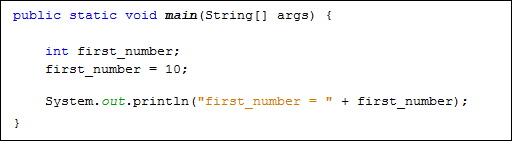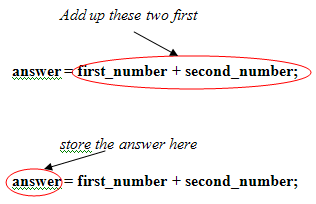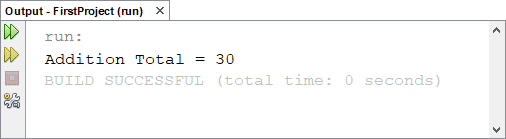Java Variables

Programmes work by manipulating data placed in memory. The data can be numbers, text, objects, pointers to other memory areas, and more besides. The data is given a name, so that it can be re-called whenever it is need. The name, and its value, is known as a Variable. We'll start with number values.

To store a number in java, you have lots of options. Whole numbers such as 8, 10, 12, etc, are stored using the int variable. (The int stands for integer.) Floating point numbers like 8.4, 10.5, 12.8, etc, are stored using the double variable. You do the storing with an equals sign ( = ). Let's look at some examples (You can use your FirstProject code for these examples).

To set up a whole number (integer), add the following to the main method of your project from the previous section:

public static void main(String[ ] args) {

int first_number;

System.out.println("My First Project");

}

So to tell Java that you want to store a whole number, you first type the word int, followed by a space. You then need to come up with a name for your integer variable. You can call them almost anything you like, but there are a few rules:

• Variable names can't start with a number. So first_number is OK, but not 1st_number. You can have numbers elsewhere in the variable name, just not at the start.
• Variable names can't be the same as Java keywords. There are quite a lot of these, and they will turn blue in NetBeans, like int above.
• You can't have spaces in your variable names. The variable declaration int first number will get you an error. We've used the underscore character, but it's common practise to have the first word start with a lowercase letter and the second or subsequent words in uppercase: firstNumber, myFirstNumber
• Variable names are case sensitive. So firstNumber and FirstNumber are different variable names.

To store something in the variable called first_number, you type an equals sign and then the value you want to store:

public static void main(String[ ] args) {

int first_number;

first_number = 10;

System.out.println("My First Project");

}

So this tells java that we want to store a value of 10 in the integer variable that we've called first_number.

If you prefer, you can do all this on one line:

public static void main(String[ ] args) {

int first_number = 10;

System.out.println("My First Project");

}

To see all this in action, change the println method slightly to this:

System.out.println( "First number = " + first_number );

What we now have between the round brackets of println is some direct text enclosed in double quotes:

("First number = "

We also have a plus sign, followed by the name of our variable:

+ first_number );

The plus sign means "join together". So we're joining together the direct text and the name of our variable. The joining together is known as concatenation.

Your coding window should now look like this (note how each line of code ends with a semicolon):Run your programme and you should see the following in the Output window at the bottom:So the number that we stored in the variable called first_number is output after the equals sign.

Let's try some simple addition. Add two more int variables to your code, one to store a second number, and one to store an answer:

Notice how we have three variable names on the same line. You can do this in Java, if the variables are of the same type (the int type, for us). Each variable name is then separated by a comma.

We can then store something in the new variables:

first_number = 10;
second_number = 20;

For the answer variable, we want to add the first number to the second number. Addition is done with the plus ( + ) symbol. Java will then add up the values in first_number and second_number. When it's finished it will store the total in the variable on the left of the equals sign. So instead of assigning 10 or 20 to the variable name, it will add up and then do the assigning. In case that's not clear, here's what happens:The above is equivalent to this:

But Java already knows what is inside of the two variables, first_number and second_number, so you can just use the names.

Now change your println method slightly to this:

Again, we are combining direct text surrounded by double quotes with a variable name. Your coding window should look like this:When you run your programme you should get the following in the output window:So our programme has done the following:

• Stored one number
• Stored a second number
• Added these two numbers together
• Stored the result of the addition in a third variable
• Printed out the result

You can also use numbers directly. Change the answer line to this:

answer = first_number + second_number + 12;

Run your programme again. What printed out? Was it what you expected?

You can store quite large numbers in the int variable type. The maximum value is 2147483647. If you want a minus number the lowest value you can have is -2147483648. If you want larger or smaller numbers you can use another number variable type: double. You'll meet them in the next part of the course.

Back to the Java Contents Page